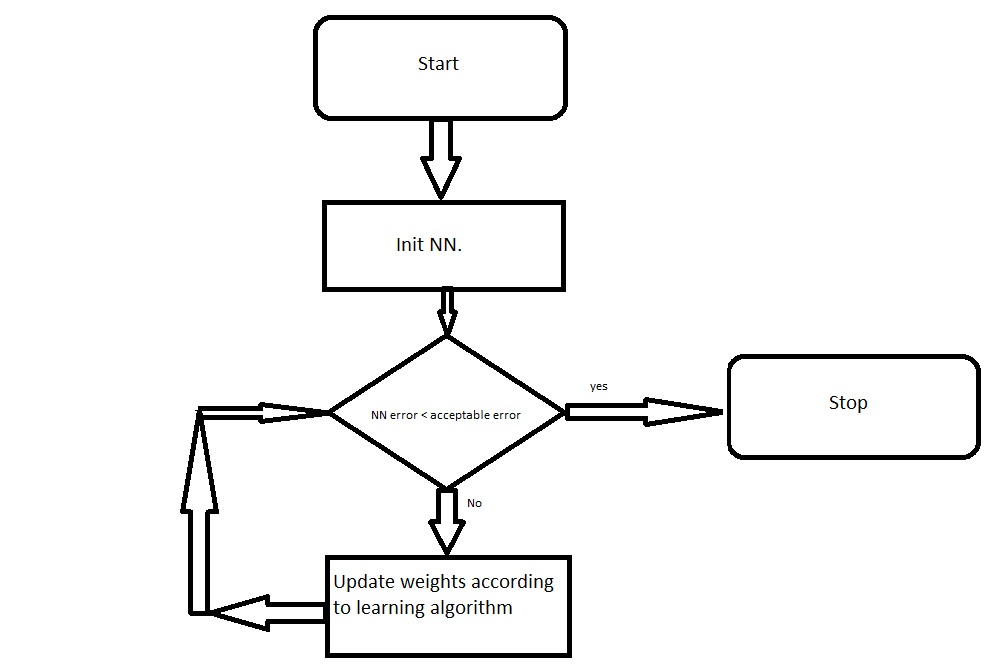Hello everybody,

today I want to describe in simple words some training algos of Encog.

Before I'll continue, I want to show general block schema of training algorithms:Init NN can look like this:

```public BasicNetwork CreateNetwork()
{
var network = new BasicNetwork();
network.Structure.FinalizeStructure();
network.Reset();
return network;
}```

Steps "NN error < acceptable error" -> "Update weights according to learning algorithim" can look like this:

```public void Train(BasicNetwork network, IMLDataSet training)
{
ITrain train = new ResilientPropagation(network, training);
int epoch = 1;
do{
train.Iteration();
Console.WriteLine(@"Epoch #" + epoch + @" Error:" + train.Error);
epoch++;
} while (train.Error > acceptableError);
}```

The first in my decription goes Backpropogation algorithm. Few characteristics of it:

1. probably the oldest training algorithm

2. As name says error are propogated back

3.  usage:

`var train = new Backpropogation(network, trainingSet, learningRate, momentum);`

The next learning algorithm goes Manhattan update rule. Few characteristics of it:

1. use only sign of the gradient

2. Updates weiht by constant value ( depending from the sign it will be added or subtracted from the weithg )

3.  usage:

`var train = new ManhattanPropogation(network, trainingSet, constantValue);`

as usually constantValue is very low ( around 0.00001 )

Other one is Quck propogation algorithm. Features of this method:

1. Newton's method of error minimization

2. Use learning trate which is higher value ( I used values starting from 2 )

3. Usage:

`var train = new QuickPropogation(network, trainingSet, learningRate);`

Yet one more training algorithm is Resilent propogation algorithm. Some details of this method:

1. One of the fastest algorithm in Encog.

2. Easiest usage ( you don't care about rate, momentum or constant value )

3. It uses sign of gradient value.

4. It tries to compute delta value for each weight and use it for calculated weight.

5.  Example of usage:

`var train = new ResilentPropogation(network, trainingSet);`

6. It has four variants of resilent propogation: RPROP+, RPROP-, iRPROP+, iRPROP- . The best is considered iRPROP+

One more detail goes Scaled conjugate gradient. Features of it:

2. Not applicable to all dataset

3. Doesn't require any learning paramethers

4. Example of usage:

`var train = new ScaledConjugateGradient(network, trainingSet);`

And the last one propogation algorithm is Levenberg Marquardt Algorithm ( aka LMA ). This is my favorite method. Features of it:

1. Something in between Gauss-Newton Algorithm ( GNA ) & Gradient Method

2. Easy to use ( no learning parameters )

3. Example of creating:

`var train = new LevenbergMarquardtTraining(network, trainingSet);`

4. Sometime it can be very efficient.